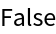Function Repository Resource:

AllSameBy

Check if all the elements of a list give the same value after applying a function

Contributed by: Sander Huisman
 ResourceFunction["AllSameBy"][list,f] gives True when all elements of the list returns the same value when f is applied, and False otherwise.

Details

ResourceFunction["AllSameBy"] applies f sequentially from the first element to the last, until the result is different from the ones before; i.e., it short-circuits whenever possible.
When list has length 0 or 1, True is automatically returned, without f being applied.

Examples

Basic Examples (1)

Test whether all numbers have the same parity:

 In:=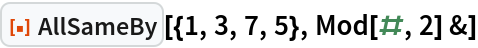Out=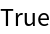Scope (1)

Test if all the numbers have the same magnitude:

 In:=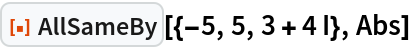Out=Applications (1)

Check if numbers are all prime or all composite:

 In:=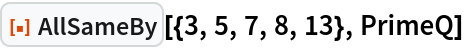Out=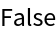Properties and Relations (4)

AllSameBy can be implemented by applying f to all elements and then checking whether the results are all the same:

 In:=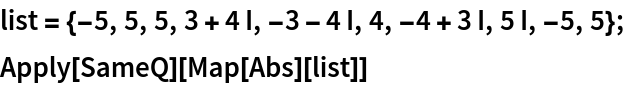Out=Compare with the original function:

 In:=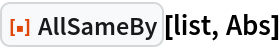Out=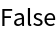The difference becomes apparent when there are side effects:

 In:=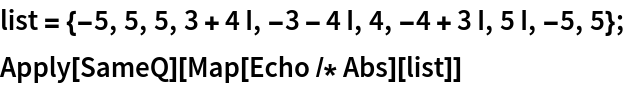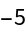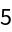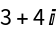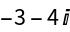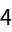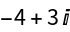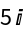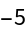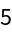Out=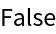Compared to the original function, the last items are untouched:

 In:=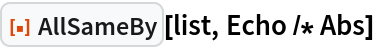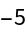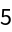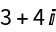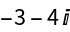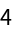Out=